[email protected] +1 203-349-9909 / +91-8080042523

# How to make Data Table in Excel: One-variable data table and Two-variable data table

Before start learning how to make a Data Table, it is essential to learn the basic about Data Table and when we can use Data Table.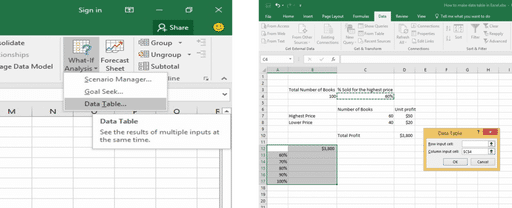## What is a Data Table?

A range of cells in where values can be changed in some of the cells and use of the formula to get different answers to a problem. Data tables are part of a suite of commands that are called what-if analysis tools. When you use data tables, you are doing what-if analysis. What-if analysis is the process of changing the values in cells to see how those changes will affect the outcome of formulas on the worksheet.

### Types of Data Table:

There are two types of Data Table based on the number of variables and formulas that you want to test.

• One-variable data table
• Two-variable data table

### When and Why?

Instead of creating a different scenario, you can create a data table to quickly try out different values for formulas. You can create a one-variable data table or a two-variable data table.

Think: you can use a data table to vary the interest rate and term length that are used in a loan to determine possible monthly payment amounts.

Case Study: Assume you own a book store and have 100 books in storage. You sell a certain % for the highest price of \$50 and a certain % for the lower price of \$20. If you sell 60% for the highest price, cell D10 below calculates a total profit of 60 * \$50 + 40 * \$20 = \$3800.

Let’s create both One-variable data table and Two-Variable data table for this case study.

## One-Variable Data Table in Excel

To create a One-variable data table, let’s execute the following steps: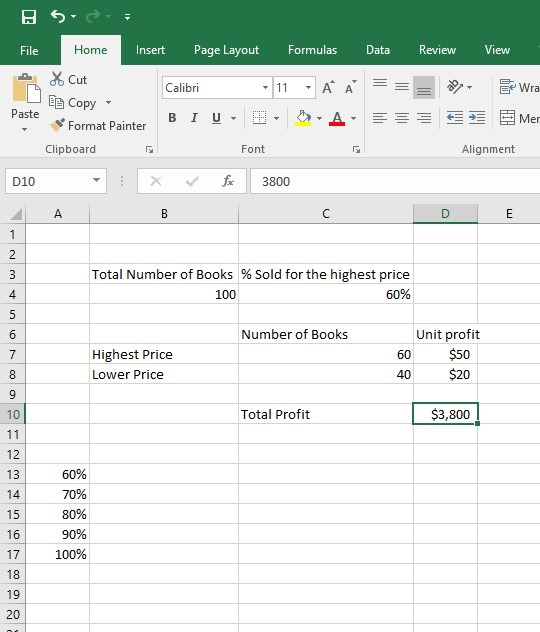Step 1: Create a table of Book sale same as like above image. Value of the cell D10 is showing the total profit. In column A from cell A13 to A17 are showing different percentages. Range B6:D8 is the table of Number of Book with Unit profit.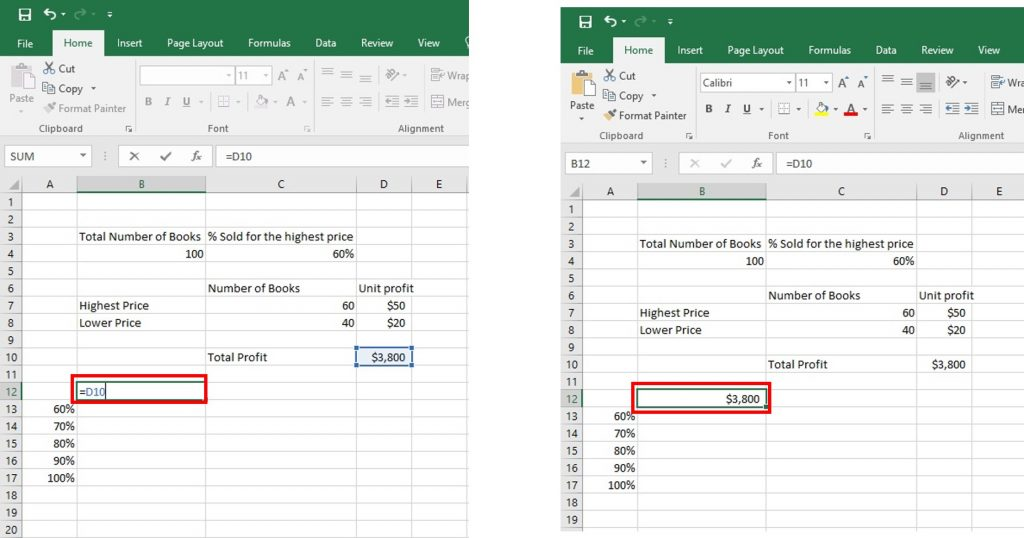Step 2: Select cell B12 and type =D10 (Refer to the total profit cell). Press Enter. Now Cell B12 is showing the value of profit cell \$3800.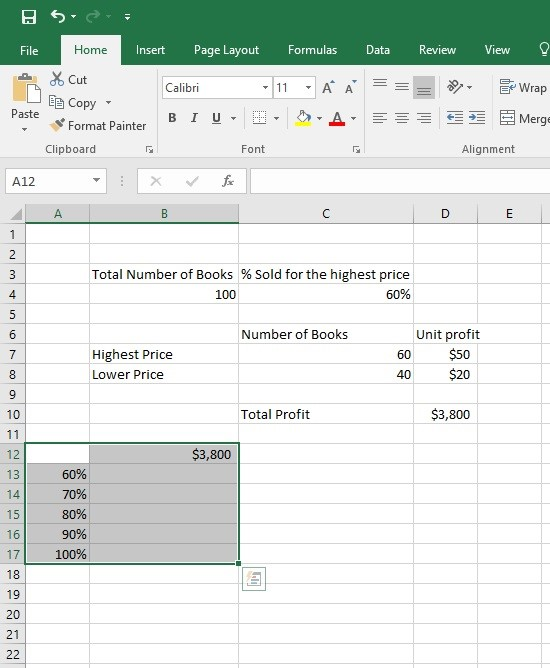Step 3: Select the range A12:B17.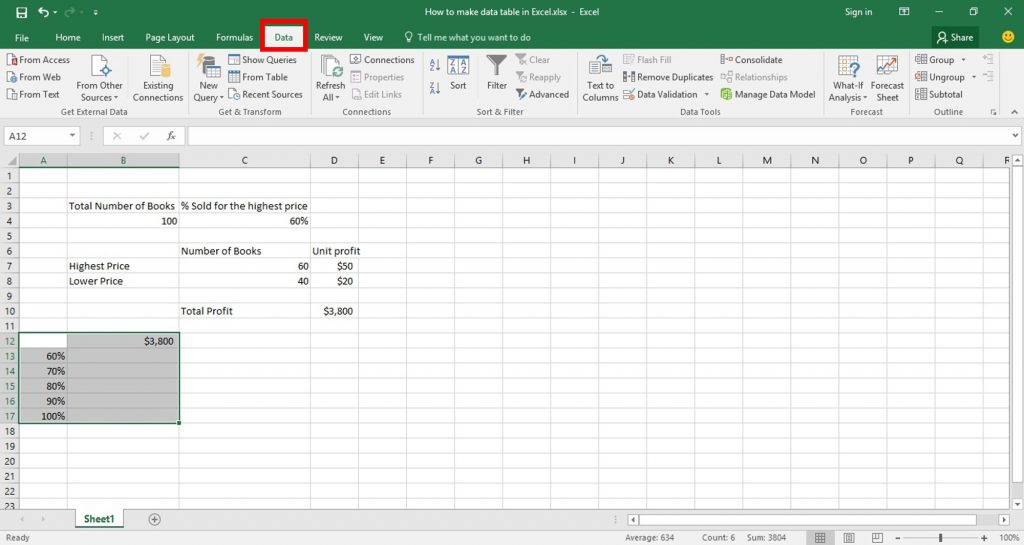Step 4: Click on Data Tab.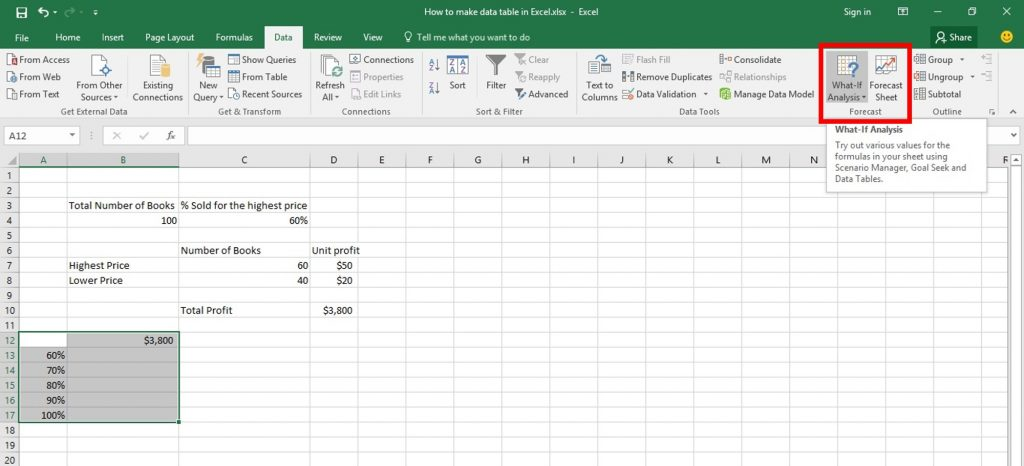Step 5: Click on What-If Analysis from Forecast Group.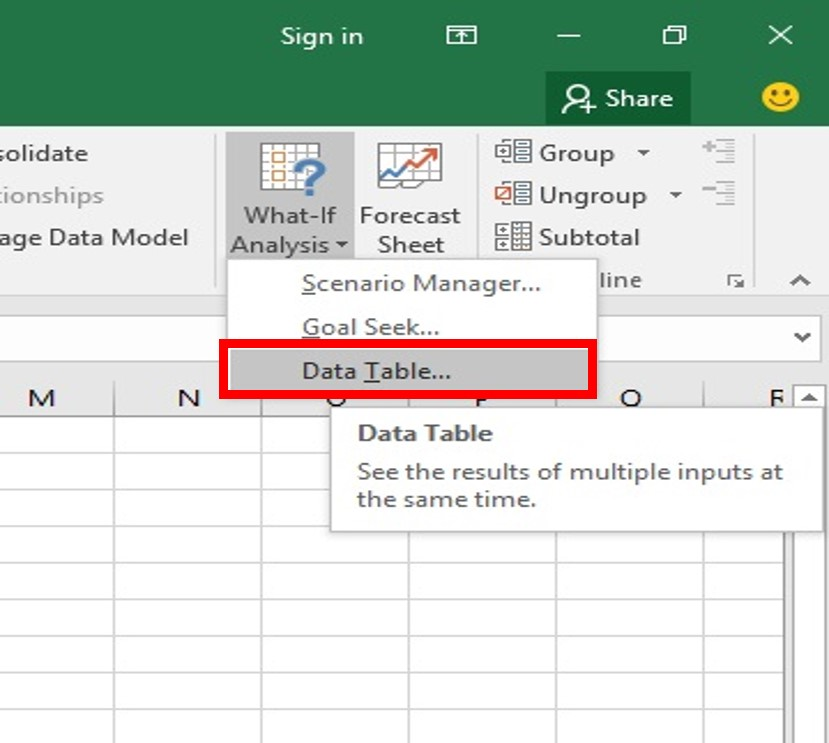Step 6: Select Data Table from the list of What-If Analysis.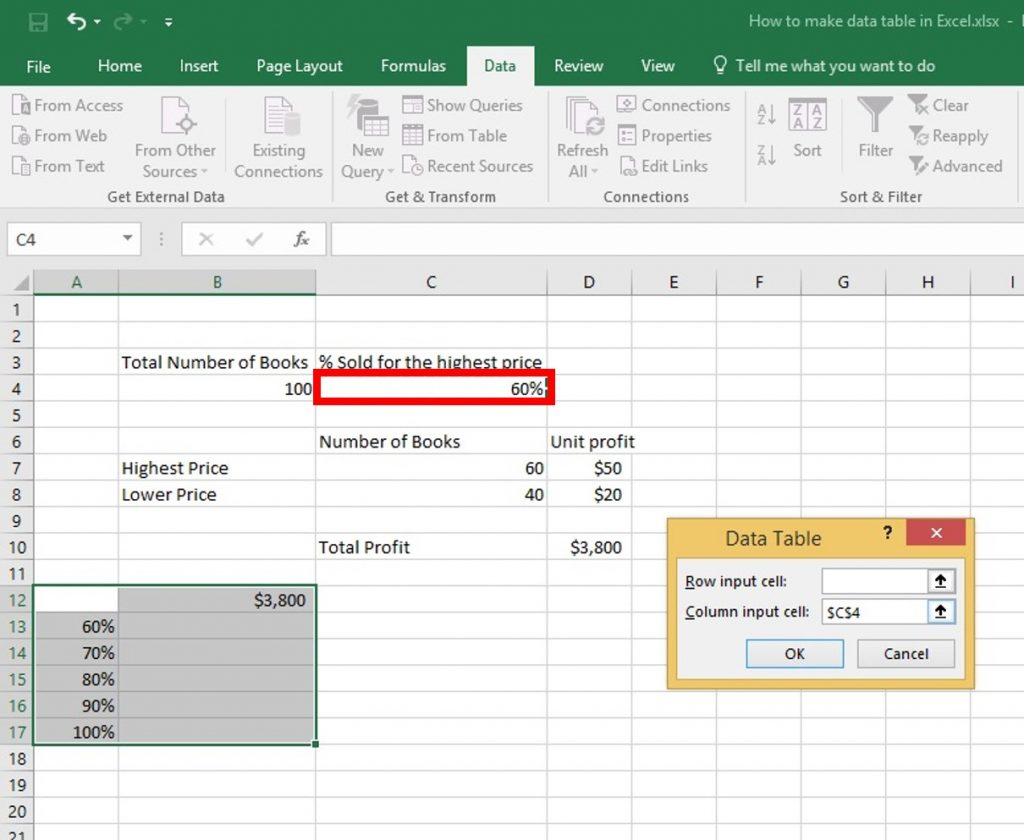Step 7: Then Data Table Dialog Box will be appeared.  Click in the Column input cell box (the percentages are in a column) and select cell C4. This is a one variable data table so we leave the Row input cell blank.

We have selected cell C4 because the percentages refer to cell C4 (% sold for the highest price). Together with the formula in cell B12, Excel now knows that it should replace cell C4 with 60% to calculate the total profit, replace cell C4 with 70% to calculate the total profit, etc.

Step 8: Click OK.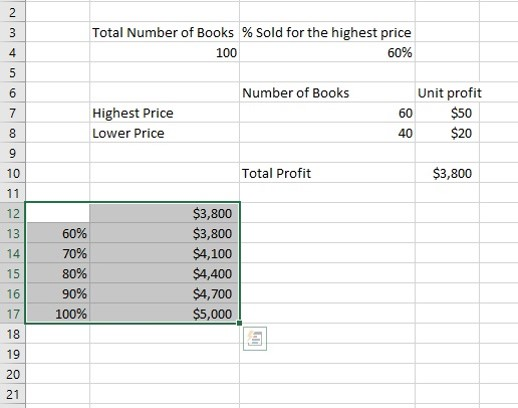Now the range A12:B17 is showing some new values from B13 to B17.  if you sell 60% for the highest price, you obtain a total profit of \$3800, if you sell 70% for the highest price, you obtain a total profit of \$4100, etc.

This is how One Variable Data table works, Now lets learn how to use Two-Variable data table

## Two-Variable Data Table

To create a Two-variable data table, let’s go through the following steps: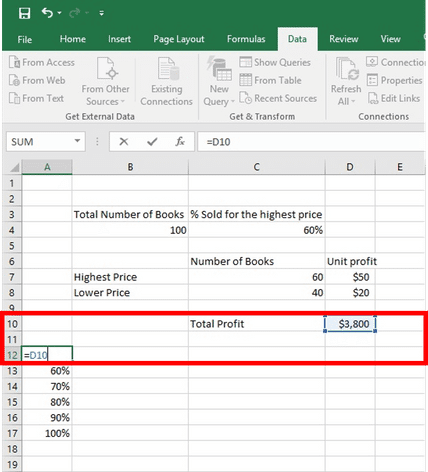Step 1: Delete values from B12 to B17. Select cell A12 and type =D10 (refer to the total profit cell).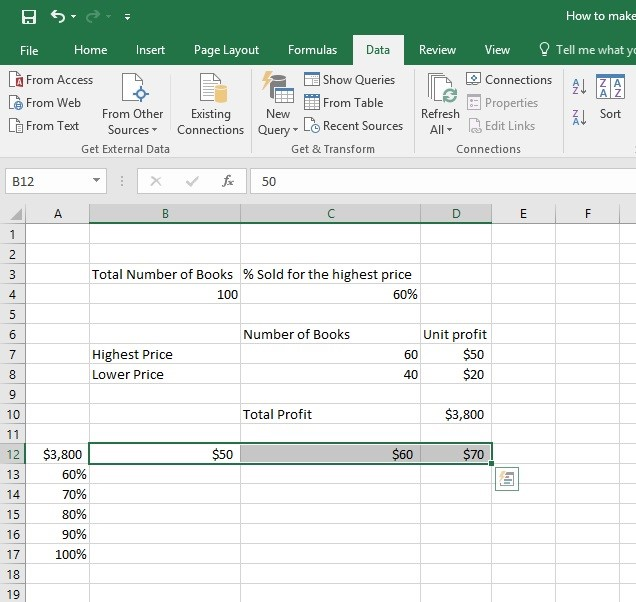Step 2: Type the different unit profits (highest price) in row 12 from B12 to D12.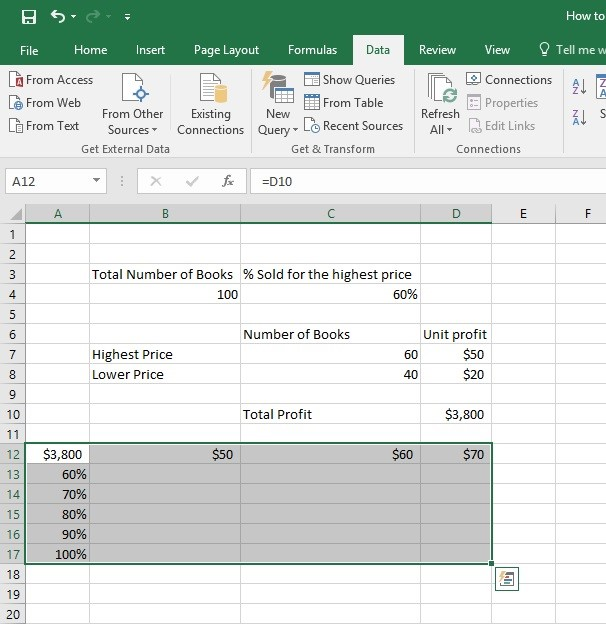Step 3: Select the range A12:D17.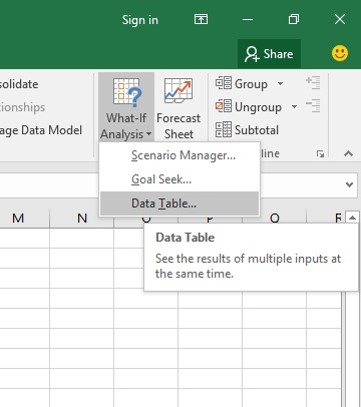Step 4: On the Data tab, click What-If Analysis and select Data Table from the list.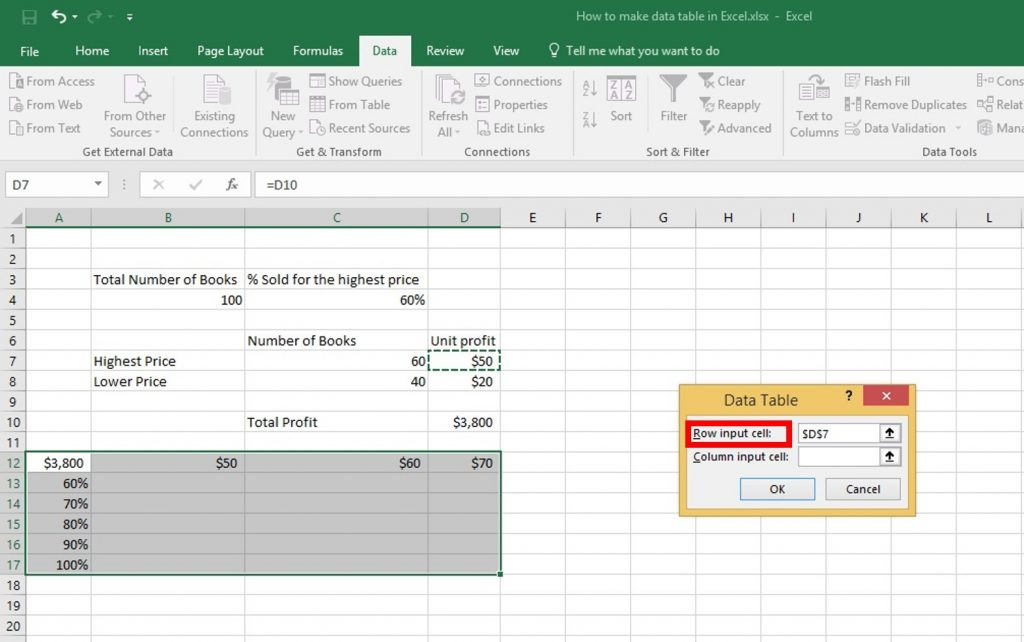Step 5: Click inside the Row input cell box (the unit profits are in a row) and select cell D7. We have selected cell D7 because the unit profits refer to cell D7.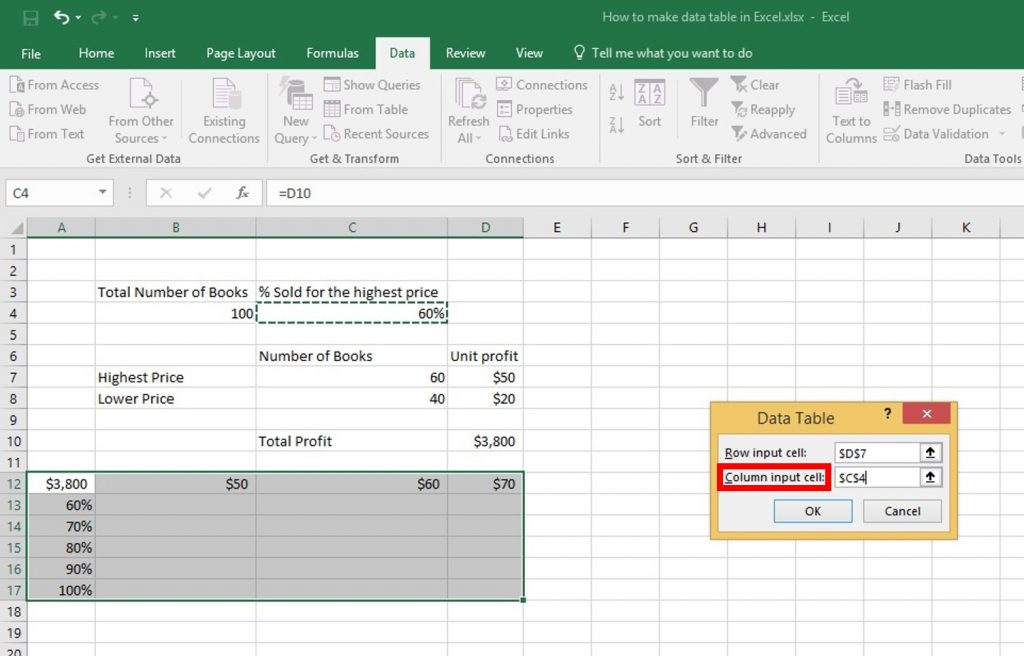Step 6: Click inside the Column input cell box (the percentages are in a column) and select cell C4. We have selected cell C4 because the percentages refer to cell C4.

Together with the formula in cell A12, Excel now knows that it should replace cell D7 with \$50 and cell C4 with 60% to calculate the total profit, replace cell D7 with \$50 and cell C4 with 70% to calculate the total profit, etc.

Step 7: Click OK.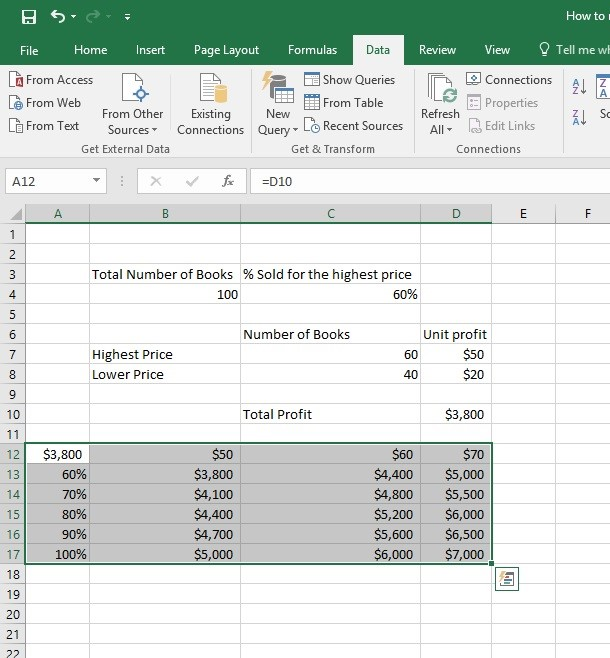If you sell 60% for the highest price, at a unit profit of \$50, you obtain a total profit of \$3800, if you sell 80% for the highest price, at a unit profit of \$60, you obtain a total profit of \$5200, etc. The formula bar indicates that the cells contain an array formula. Therefore, you cannot delete a single result. To delete the results, select the range B13:D17 and press Delete.

Now You have learned How to create Data Table Successfully..!!